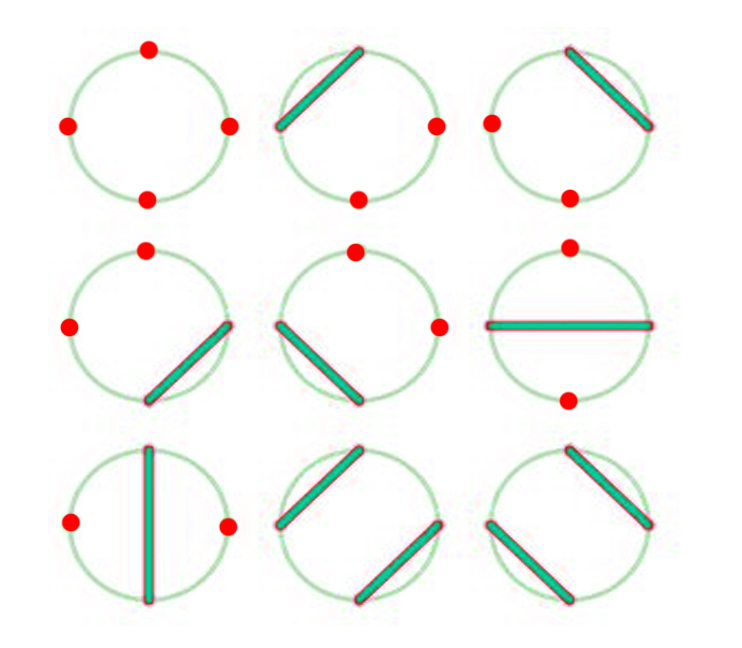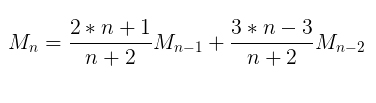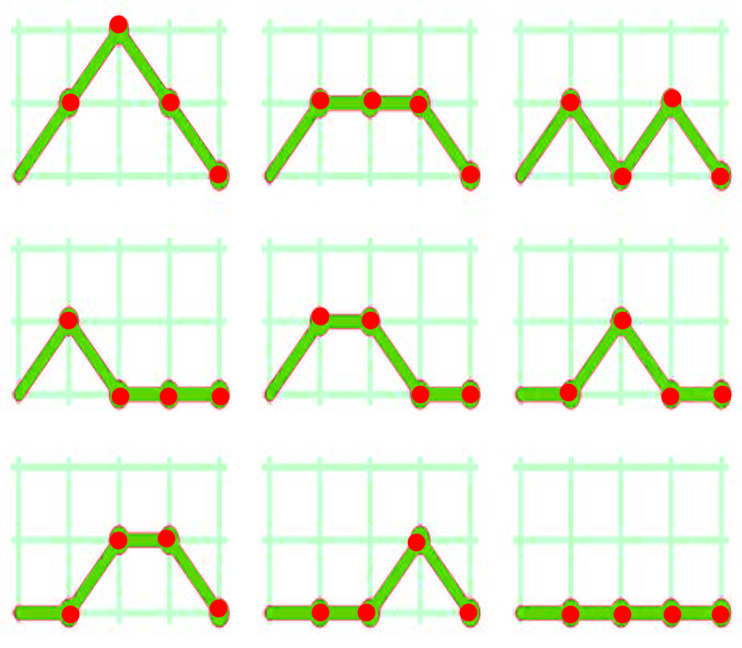# Motzkin number

In mathematics, a Motzkin number for a given number n is the number of different ways of drawing non-intersecting chords between n points on a circle (not necessarily touching every point by a chord).
For example, for n = 3, M4 = 9.Recurrence relation for Nth Motzkin Number is :Motzkin Number can be used to find :

• Number of positive integer sequences of length n – 1 in which the opening and ending elements are either 1 or 2, and the difference between any two consecutive elements is -1, 0 or 1.
• Number of routes on the upper right quadrant of a grid from coordinate (0, 0) to coordinate (n, 0) in n steps if one is allowed to move only to the right (up, down or straight) at each step but forbidden from dipping below the y = 0 axis.
• For example –

Following figure shows the 9 valid Motzkin paths from (0, 0) to (4, 0).Examples :

```Input : n = 4
Output : 9

Input : n = 5
Output : 21
```

## Recommended: Please try your approach on {IDE} first, before moving on to the solution.

Below is the program to find nth Motzkin Number :

## C++

 `// CPP Program to find Nth Motzkin Number. ` `#include ` `using` `namespace` `std; ` ` `  `// Return the nth Motzkin Number. ` `int` `motzkin(``int` `n) ` `{ ` `    ``// Base Case ` `    ``if` `(n == 0 || n == 1) ` `        ``return` `1; ` ` `  `    ``// Recursive step ` `    ``return` `((2 * n + 1) * motzkin(n - 1) + ` `            ``(3 * n - 3) * motzkin(n - 2)) / (n + 2); ` `} ` ` `  `// Driven Program ` `int` `main() ` `{ ` `    ``int` `n = 8; ` `    ``cout << motzkin(n) << endl; ` `    ``return` `0; ` `} `

## Java

 `// Java Program to find Nth Motzkin Number. ` `import` `java.util.*; ` ` `  `class` `Digits ` `{ ` `    ``// Return the nth Motzkin Number. ` `    ``public` `static` `int` `motzkin(``int` `n) ` `    ``{ ` `        ``// Base Case ` `        ``if` `(n == ``0` `|| n == ``1``) ` `            ``return` `1``; ` ` `  `        ``// Recursive step ` `        ``return` `((``2` `* n + ``1``) * motzkin(n - ``1``) + ` `                ``(``3` `* n - ``3``) * motzkin(n - ``2``)) / (n + ``2``); ` `    ``}  ` `     `  `    ``// driver code     ` `    ``public` `static` `void` `main(String[] args) ` `    ``{ ` `        ``int` `n = ``8``; ` `        ``System.out.print( motzkin(n) ); ` `    ``} ` `} ` ` `  `// This code is contributed by rishabh_jain `

## Python3

 `# Python3 program to find Nth Motzkin Number. ` ` `  `# Return the nth Motzkin Number. ` `def` `motzkin(n) : ` `     `  `    ``# Base Case ` `    ``if` `(n ``=``=` `0` `or` `n ``=``=` `1``) : ` `        ``return` `1` ` `  `    ``# Recursive step ` `    ``return` `((``2` `*` `n ``+` `1``) ``*` `motzkin(n ``-` `1``) ``+`  `            ``(``3` `*` `n ``-` `3``) ``*` `motzkin(n ``-` `2``)) ``/` `(n ``+` `2``) ` ` `  `# Driver code ` `n ``=` `8` `print``( motzkin(n) ) ` ` `  `# This code is contributed by rishabh_jain `

## C#

 `// C# Program to find Nth Motzkin Number. ` `using` `System; ` ` `  `class` `GFG { ` `     `  `    ``// Return the nth Motzkin Number. ` `    ``public` `static` `int` `motzkin(``int` `n) ` `    ``{ ` `         `  `        ``// Base Case ` `        ``if` `(n == 0 || n == 1) ` `            ``return` `1; ` ` `  `        ``// Recursive step ` `        ``return` `((2 * n + 1) * motzkin(n - 1) + ` `            ``(3 * n - 3) * motzkin(n - 2)) / (n + 2); ` `    ``}  ` `     `  `    ``// driver code  ` `    ``public` `static` `void` `Main() ` `    ``{ ` `        ``int` `n = 8; ` `         `  `        ``Console.WriteLine( motzkin(n) ); ` `    ``} ` `} ` ` `  `// This code is contributed by vt_m `

## PHP

 ` `

Output :

```323
```

Using Dynamic Programming :

Below is the Dynamic Programming solution of finding nth Motzkin Number :

## C++

 `// CPP Program to find Nth Motzkin Number. ` `#include ` `using` `namespace` `std; ` ` `  `// Return the nth Motzkin Number. ` `int` `motzkin(``int` `n) ` `{ ` `    ``int` `dp[n + 1]; ` ` `  `    ``// Base case ` `    ``dp = dp = 1; ` ` `  `    ``// Finding i-th Motzkin number. ` `    ``for` `(``int` `i = 2; i <= n; i++) ` `        ``dp[i] = ((2 * i + 1) * dp[i - 1] +  ` `                  ``(3 * i - 3) * dp[i - 2]) / (i + 2); ` ` `  `    ``return` `dp[n]; ` `} ` `// Driven Program ` `int` `main() ` `{ ` `    ``int` `n = 8; ` `    ``cout << motzkin(n) << endl; ` `    ``return` `0; ` `} `

## Java

 `// Java Program to find Nth Motzkin Number. ` `import` `java.util.*; ` ` `  `class` `Digits ` `{ ` `    ``// Return the nth Motzkin Number. ` `    ``public` `static` `int` `motzkin(``int` `n) ` `    ``{ ` `        ``int``[] dp = ``new` `int``[n+``1``]; ` ` `  `        ``// Base case ` `        ``dp[``0``] = dp[``1``] = ``1``; ` ` `  `        ``// Finding i-th Motzkin number. ` `        ``for` `(``int` `i = ``2``; i <= n; i++) ` `            ``dp[i] = ((``2` `* i + ``1``) * dp[i - ``1``] +  ` `                ``(``3` `* i - ``3``) * dp[i - ``2``]) / (i + ``2``); ` ` `  `        ``return` `dp[n]; ` `    ``} ` `     `  `    ``// driver code     ` `    ``public` `static` `void` `main(String[] args) ` `    ``{ ` `        ``int` `n = ``8``; ` `        ``System.out.print( motzkin(n) ); ` `    ``} ` `} ` ` `  `// This code is contributed by rishabh_jain `

## Python3

 `# Python3 program to find Nth Motzkin Number. ` ` `  `# Return the nth Motzkin Number. ` `def` `motzkin(n) : ` `     `  `    ``dp ``=` `[``None``] ``*` `(n``+``1``) ` ` `  `    ``# Base case ` `    ``dp[``0``] ``=` `dp[``1``] ``=` `1``; ` ` `  `    ``i ``=` `2` `    ``# Finding i-th Motzkin number. ` `    ``while` `i <``=` `n : ` `        ``dp[i] ``=` `((``2` `*` `i ``+` `1``) ``*` `dp[i ``-` `1``] ``+`  `                ``(``3` `*` `i ``-` `3``) ``*` `dp[i ``-` `2``]) ``/` `(i ``+` `2``); ` `        ``i ``=` `i ``+` `1` `    ``return` `dp[n]; ` ` `  `# Driver code ` `n ``=` `8` `print``( motzkin(n) ) ` ` `  `# This code is contributed by rishabh_jain `

## C#

 `// C# Program to find Nth Motzkin Number. ` `using` `System; ` ` `  `class` `GFG { ` `     `  `    ``// Return the nth Motzkin Number. ` `    ``public` `static` `int` `motzkin(``int` `n) ` `    ``{ ` `        ``int``[] dp = ``new` `int``[n+1]; ` ` `  `        ``// Base case ` `        ``dp = dp = 1; ` ` `  `        ``// Finding i-th Motzkin number. ` `        ``for` `(``int` `i = 2; i <= n; i++) ` `            ``dp[i] = ((2 * i + 1) * dp[i - 1] +  ` `             ``(3 * i - 3) * dp[i - 2]) / (i + 2); ` ` `  `        ``return` `dp[n]; ` `    ``} ` `     `  `    ``// driver code  ` `    ``public` `static` `void` `Main() ` `    ``{ ` `        ``int` `n = 8; ` `         `  `        ``Console.WriteLine( motzkin(n) ); ` `    ``} ` `} ` ` `  `// This code is contributed by vt_m `

## PHP

 ` `

Output :

```323
```

Attention reader! Don’t stop learning now. Get hold of all the important DSA concepts with the DSA Self Paced Course at a student-friendly price and become industry ready.

My Personal Notes arrow_drop_upCheck out this Author's contributed articles.

If you like GeeksforGeeks and would like to contribute, you can also write an article using contribute.geeksforgeeks.org or mail your article to contribute@geeksforgeeks.org. See your article appearing on the GeeksforGeeks main page and help other Geeks.

Please Improve this article if you find anything incorrect by clicking on the "Improve Article" button below.

Improved By : jit_t

Article Tags :
Practice Tags :

Be the First to upvote.

Please write to us at contribute@geeksforgeeks.org to report any issue with the above content.